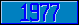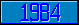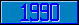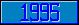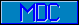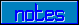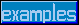## Arithmetic operator 'modulo' (#)

Introduced in the 1977 ANSI M[UMPS] language standard.

This is probably the most mis-understood operator (or function) in many programming languages. FORTRAN and C have 'remainder' operators, Ada and M[UMPS] have a true 'modulo' operator. These two are not the same (although the result is identical when both operands are positive).

The definition of remainder depends on where you went to school. If you learned math in Europe, you will have a radically different opinion about the sign of a remainder than if you went to school in the USA (when either or both of the operands are negative, that is, when both operands are positive, everyone agrees).
Both 'schools' are correct, and, as long as you follow your definition consistently, you will have no problems.
In M[UMPS], however, there is no remainder operator. There is the modulo instead.
The definition of modulo is based on Abelian group theory, and the true mathematical definition is little known outside of mathematical graduate schools (see for instance Donald Knuth's The Art of Computer Programming, Volume 1, page 23 and further), but the following explanation is close enough for practical purposes:

The mathematical definition of Modulo maps a potentially infinitely large set of numbers onto a (usually finite) subset. The subset is repeated cyclically to map every number from the original set onto a member of the subset.
number#sub means: map the number on the left-hand side onto the subset [0,sub) (0, zero inclusive, sub exclusive). If sub is negative, this should be (sub,0], of course.
For example, number#5 and number#-5 would map as follows:

 number -9 -8 -7 -6 -5 -4 -3 -2 -1 0 1 2 3 4 5 6 7 8 9 number#(5) 1 2 3 4 0 1 2 3 4 0 1 2 3 4 0 1 2 3 4 number#(-5) -4 -3 -2 -1 0 -4 -3 -2 -1 0 -4 -3 -2 -1 0 -4 -3 -2 -1

Added in the 1995 ANSI M[UMPS] language standard:

Reference   Value
X#0   Error (M9)